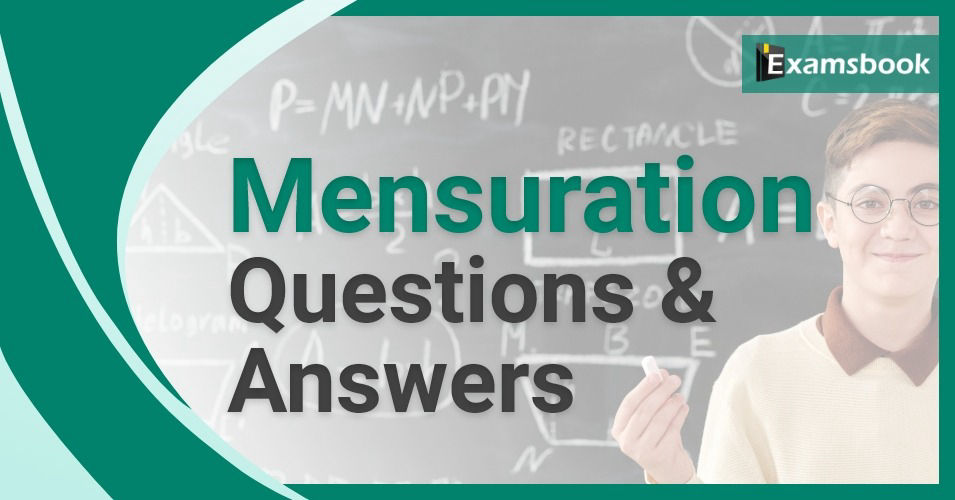• Save

# Mensuration Questions and Answers for Competitive Exams

Gajanand8 months ago 39.0K ViewsMensuration topic is an important topic in the aptitude section. Students can start their practice with mensuration questions and answers who are preparing for competitive exams. You can get the important questions related to this topic which will help you to increase your score in the competitive exams.

Q :  Length of a rectangle is 53 metre, while its breath is 28 metre. Cost of covering it with grass bed is Rs.27 sq meter. Find total expenditure?

(A) Rs. 40,098

(B) Rs. 40,048

(C) Rs. 40,058

(D) Rs. 40,088

(E) None of these

Q :  Base of a right-angle triangle is 9 cm and its area is 81 sq cm. Find its height.

(A) 36 cm

(B) 9 cm

(C) 27 cm

(D) None of these

Q :  Difference between the circumferences of two circles is 132 cm and radius of the smaller circle is 14 cm. Find the radius of the larger circle?

(A) 14 cm

(B) 21 cm

(C) 30 cm

(D) None of these

Q :  Poles are to be created along the boundary of a rectangular field in such a way that distance between any two adjacent poles is 1.5 metres. The perimeter of the field is 21 metres and length and the breadth are in the ratio of 4:3 respectively. How many poles will be required?

(A) 14

(B) 16

(C) 15

(D) 20

Q :  The ratio of length and breadth of rectangle is 5:2 respectively. The respective ratio of its perimeter and area is 1:3 (irrespective of the unit). What is the length of the rectangle?

(A) 27 units

(B) 32 units

(C) 21 units

(D) None of these

Q :  The length of a rectangle, which is 25 cm is equal to the length of a square and the area of the rectangle is 125 square cm less than the area of the square. What is the breadth of the rectangle?

(A) 15 cm

(B) 20 cm

(C) 12 cm

(D) 14 cm

Q :  The area of a rectangle with 10 cm length is equal to the area of a circle. Find the circumference of the circle.

(A) 36 cm

(B) 34 cm

(C) 26 cm

(D) cannot be determined

Q :  If the length of a rectangle is increased by 20% and the breadth is decreased by 10%. What will be the effect on its area?

(A) 8% increase

(B) 8% decrease

(C) 2% increase

(D) 2% decrease

Q :  The ratio of length and breadth of a rectangular plot is 71:16 respectively. The area of the plot is 17324 sq.metres. What is the perimeter of the plot?

(A) 284 metres

(B) 528 metres

(C) 264 metres

(D) 614 metres

Q :  If area of a circular jogging track is 3850 sq.metres. What is the circumference of the jogging track?

(A) 225 metres

(B) 214 metres

(C) 220 metres

(D) 235 metres

### Gajanand

B-Tech Electronic and Communication Engineering select the SSC CGL Tier-1 and Tier-2 in 2016 and Rajasthan state Govt. Exams Expert in Mathematics , Reasoning Gk and English.